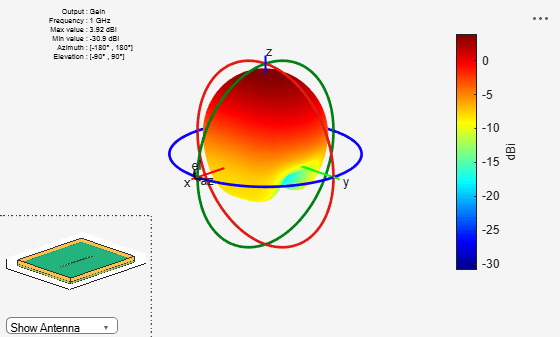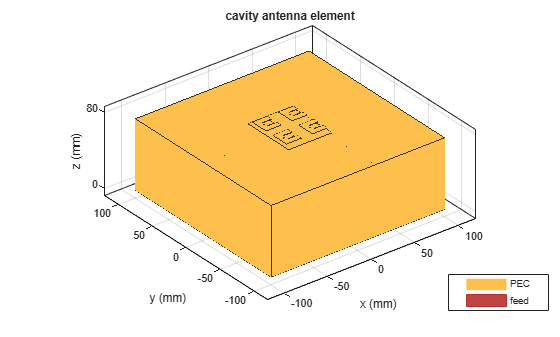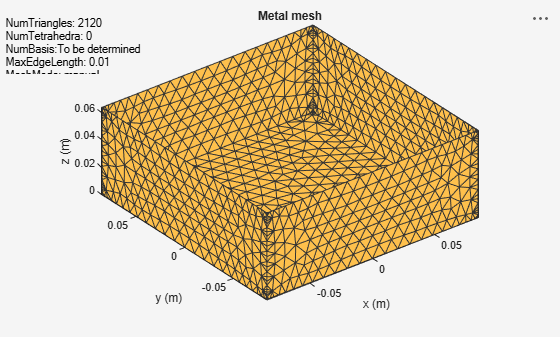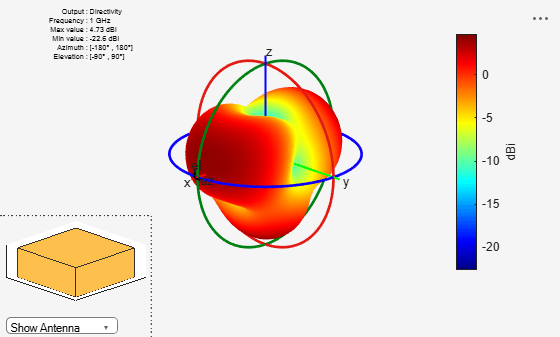# cavity

Create cavity-backed antenna

## Description

The `cavity` object is a cavity-backed antenna located on the xyz- plane. The default cavity antenna has a dipole as an exciter. The feed point is on the exciter.## Creation

### Syntax

``c = cavity``
``c = cavity(Name=Value)``

### Description

``` `c = cavity` creates a cavity backed antenna located on the X-Y-Z plane. By default, the dimensions are chosen for an operating frequency of 1 GHz.```

example

``` `c = cavity(Name=Value)` creates a cavity-backed antenna, with additional Properties specified by one or more name–value arguments. `Name` is the property name and `Value` is the corresponding value. You can specify several name-value arguments in any order as `Name1`= `Value1`, `...`, `NameN`=`ValueN`. Properties not specified retain their default values.```

## Properties

expand all

Exciter antenna or array type, specified as a single-element antenna object, an array object, or an empty array. Except for reflector and cavity antenna elements, you can use any Antenna Toolbox™ antenna or array element as an exciter. To create the cavity backing structure without the exciter, specify this property as an empty array.

Example: `horn`

Example: `linearArray(Element=patchMicrostrip)`

Example: `[]`

Type of dielectric material used as a substrate, specified as a dielectric object. For more information see, `dielectric`. For more information on dielectric substrate meshing, see Meshing.

Note

The substrate dimensions must be equal to the ground plane dimensions.

Example: `dielectric("FR4")`

Length of the rectangular cavity along the x-axis, specified as a scalar in meters.

Example: `30e-2`

Data Types: `double`

Width of the rectangular cavity along the y-axis, specified as a scalar in meters.

Example: `25e-2`

Data Types: `double`

Height of the rectangular cavity along the z-axis, specified as a scalar in meters.

Example: `7.5e-2`

Data Types: `double`

Distance between the exciter and the base of the cavity, specified as a scalar in meters.

Example: `7.5e-2`

Data Types: `double`

Type of the metal used as a conductor, specified as a metal material object. You can choose any metal from the `MetalCatalog` or specify a metal of your choice. For more information, see `metal`. For more information on metal conductor meshing, see Meshing.

Example: `metal("Copper")`

Lumped elements added to the antenna feed, specified as a lumped element object. For more information, see `lumpedElement`.

Example: `lumpedElement(Impedance=75)`

Create probe feed from backing structure to exciter, specified as a `0` or `1`. By default, probe feed is not enabled.

Example: `1`

Data Types: `double`

Tilt angle of the antenna, specified as a scalar or vector with each element unit in degrees. For more information, see Rotate Antennas and Arrays.

Example: `Tilt=90`

Example: `Tilt=[90 90]`,`TiltAxis=[0 1 0;0 1 1]` tilts the antenna at 90 degrees about the two axes defined by the vectors.

Note

The `wireStack` antenna object only accepts the dot method to change its properties.

Data Types: `double`

Tilt axis of the antenna, specified as:

• Three-element vector of Cartesian coordinates in meters. In this case, each coordinate in the vector starts at the origin and lies along the specified points on the X-, Y-, and Z-axes.

• Two points in space, each specified as three-element vectors of Cartesian coordinates. In this case, the antenna rotates around the line joining the two points in space.

• A string input describing simple rotations around one of the principal axes, 'X', 'Y', or 'Z'.

Example: `TiltAxis=[0 1 0]`

Example: `TiltAxis=[0 0 0;0 1 0]`

Example: `TiltAxis = 'Z'`

Data Types: `double`

## Object Functions

 `show` Display antenna, array structures or shapes `info` Display information about antenna or array `axialRatio` Axial ratio of antenna `beamwidth` Beamwidth of antenna `charge` Charge distribution on antenna or array surface `current` Current distribution on antenna or array surface `design` Design prototype antenna or arrays for resonance around specified frequency `efficiency` Radiation efficiency of antenna `EHfields` Electric and magnetic fields of antennas; Embedded electric and magnetic fields of antenna element in arrays `impedance` Input impedance of antenna; scan impedance of array `mesh` Mesh properties of metal, dielectric antenna, or array structure `meshconfig` Change mesh mode of antenna structure `optimize` Optimize antenna or array using SADEA optimizer `pattern` Radiation pattern and phase of antenna or array; Embedded pattern of antenna element in array `patternAzimuth` Azimuth pattern of antenna or array `patternElevation` Elevation pattern of antenna or array `rcs` Calculate and plot radar cross section (RCS) of platform, antenna, or array `returnLoss` Return loss of antenna; scan return loss of array `sparameters` Calculate S-parameter for antenna and antenna array objects `vswr` Voltage standing wave ratio of antenna

## Examples

collapse all

Create and view a cavity-backed dipole antenna with 30 cm length, 25 cm width, 7.5 cm height and spaced 7.5 cm from the bowtie for operation at 1 GHz.

```c = cavity(Length=30e-2,Width=25e-2,Height=7.5e-2,Spacing=7.5e-2); show(c)```Create a cavity-backed antenna using a dielectric substrate 'FR4'.

```d = dielectric('FR4'); c = cavity(Length=30e-2,Width=25e-2,Height=20.5e-3,Spacing=7.5e-3,... Substrate=d)```
```c = cavity with properties: Exciter: [1x1 dipole] Substrate: [1x1 dielectric] Length: 0.3000 Width: 0.2500 Height: 0.0205 Spacing: 0.0075 EnableProbeFeed: 0 Conductor: [1x1 metal] Tilt: 0 TiltAxis: [1 0 0] Load: [1x1 lumpedElement] ```
`show(c)`Plot the radiation pattern of the antenna at a frequency of 1 GHz.

```figure pattern(c,1e9)```Create a rectangular array of E-shaped patch antenna.

`rectArr = rectangularArray(Element=patchMicrostripEnotch,RowSpacing=0.03,ColumnSpacing=0.03);`

Create a cavity-backed antenna with rectangular array exciter.

`ant = cavity(Exciter=rectArr)`
```ant = cavity with properties: Exciter: [1x1 rectangularArray] Substrate: [1x1 dielectric] Length: 0.2000 Width: 0.2000 Height: 0.0750 Spacing: 0.0750 EnableProbeFeed: 0 Conductor: [1x1 metal] Tilt: 0 TiltAxis: [1 0 0] Load: [1x1 lumpedElement] ```
`show(ant)`Create and visualize a cavity-backed fractal carpet antenna

```e = fractalCarpet(Substrate=dielectric('FR4')); ant = cavity(Exciter=e)```
```ant = cavity with properties: Exciter: [1x1 fractalCarpet] Substrate: [1x1 dielectric] Length: 0.2000 Width: 0.2000 Height: 0.0750 Spacing: 0.0750 EnableProbeFeed: 0 Conductor: [1x1 metal] Tilt: 0 TiltAxis: [1 0 0] Load: [1x1 lumpedElement] ```
`show(ant)`This example shows how to create and analyze a cavity-shaped backing structure without an exciter element using `planeWaveExcitation`.

Create Cavity Antenna

Create a cavity antenna operating at 1 GHz using the `design` function and the `cavity` element from the antenna catalog. Display the antenna.

```f = 1e9; ant = design(cavity,f); figure show(ant)```Derive Backing Structure

Derive the backing structure from the cavity antenna by specifying the `'Exciter'` property as an empty array.

`ant.Exciter = []`
```ant = cavity with properties: Exciter: [] Substrate: [1x1 dielectric] Length: 0.1690 Width: 0.1690 Height: 0.0634 Spacing: 0.0634 EnableProbeFeed: 0 Conductor: [1x1 metal] Tilt: 0 TiltAxis: [1 0 0] Load: [1x1 lumpedElement] ```

Display the backing structure.

```figure show(ant)```Mesh Backing Structure

Mesh the cavity structure with a maximum edge length of 10 mm.

```figure mesh(ant,MaxEdgeLength=10e-3)```Plot Directivity Pattern

Use the cavity backing structure as a receiver element in a plane wave excitation environment and plot its directivity at 1 GHz.

```pw = planeWaveExcitation(Element=ant); figure pattern(pw,f)```Balanis, Constantine A. Antenna Theory: Analysis and Design. Fourth edition. Hoboken, New Jersey: Wiley, 2016.